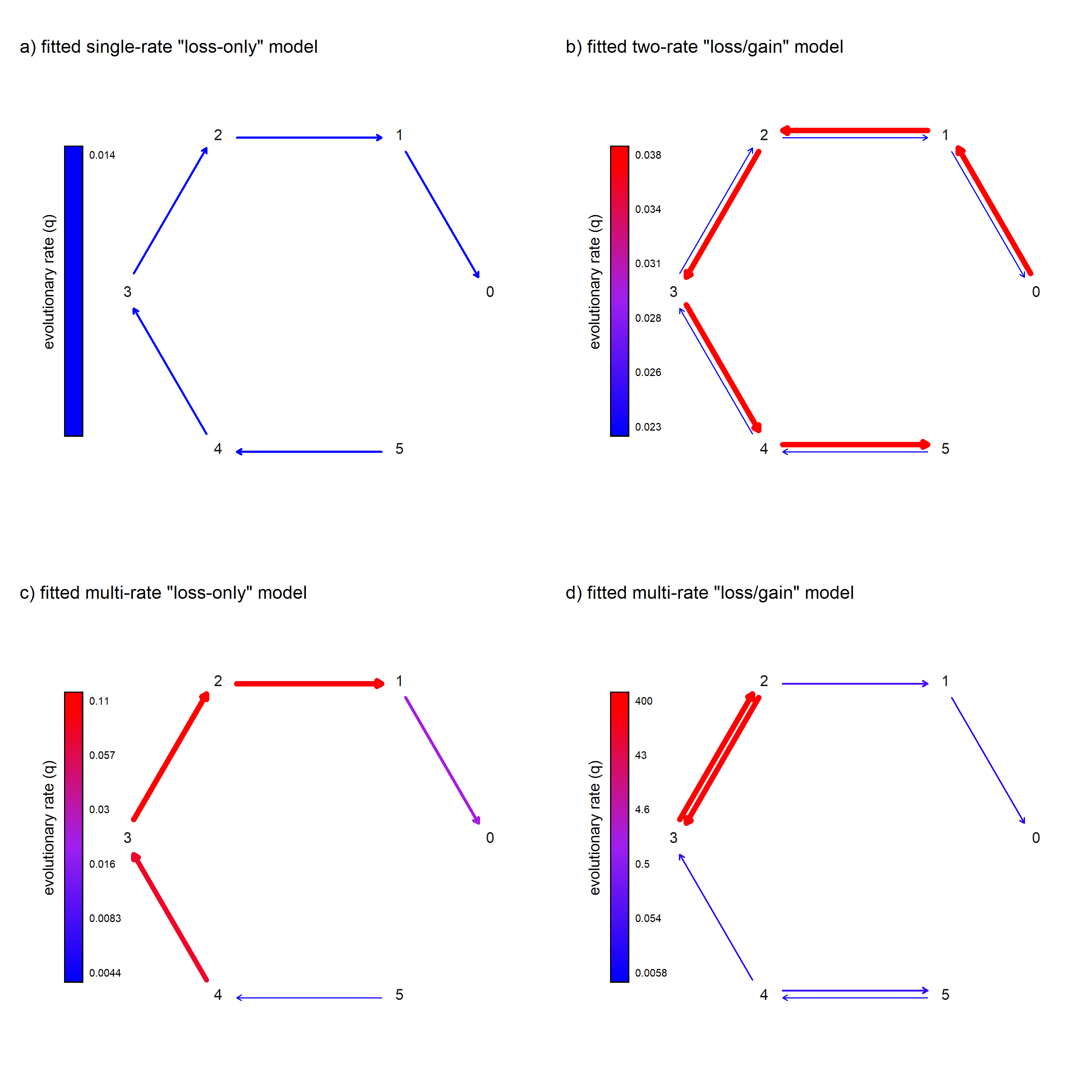## Thursday, September 1, 2022

### More updates to visualization of fitted Mk models and new `anova` method for `"fitMk"` object class

Over the last couple of days (1, 2), I've posted about plotting fitted Mk models in which we visualize rate differences among transition types using different colors and arrow widths.

These posts combined both pre-existing and totally new functionality of the phytools package. Unfortunately, these plotting methods did not make it into my book with Luke Harmon (I wish they had) – but, nonetheless!

After a few additional updates, here's a workflow that illustrates applying these new graphing methods to a phylogeny and dataset for squamate digit number evolution from Brandley et al. (2008) that can be obtained from our book website.

First, load packages. To follow along, readers will have to update phytools from GitHub, which can be done most easily using the CRAN package devtools.

``````library(phytools)
packageVersion("phytools")
``````
``````##  '1.1.17'
``````

Now let's read in our phylogeny and data from the book website.

``````## read data matrix
row.names=1)
## name.check
chk<-geiger::name.check(sqTree,sqData)
## drop tips of tree that are missing from data matrix
sqTree.pruned<-drop.tip(sqTree,chk\$tree_not_data)
## drop rows of matrix that are missing from tree
sqData.pruned<-sqData[!(rownames(sqData)%in%
chk\$data_not_tree),,drop=FALSE]
## extract trait
toes<-setNames(as.factor(sqData.pruned[,"rear.toes"]),
rownames(sqData.pruned))
``````

Terrific. Now we're ready to fit our models.

In the book we fit equal-rates (“ER”) and all-rates-different (“ARD”) models, but (spoiler alert) they don't explain our data very well.

Here, instead, I'm going to fit a series of more biologically-informed models: one of only digit loss, with a single rate; a second with digit loss and gain, but only between adjacent states, with one rate of loss and one of gain; and then the more parameter-rich directional (loss-only) and ordered (gain-and-loss) models.

To fit each of these models, I first build a design matrix indicating which types of transitions are permitted (and allowed to assume different rates). Then I pass this design matrix to `phytools::fitMk` to fit the model.

``````## loss-only (one-rate)
loss.model<-matrix(c(
0,0,0,0,0,0,
1,0,0,0,0,0,
0,1,0,0,0,0,
0,0,1,0,0,0,
0,0,0,1,0,0,
0,0,0,0,1,0),6,6,byrow=TRUE,
dimnames=list(0:5,0:5))
fitLoss<-fitMk(sqTree.pruned,toes,model=loss.model,pi="fitzjohn")
fitLoss
``````
``````## Object of class "fitMk".
##
## Fitted (or set) value of Q:
##          0         1         2         3         4         5
## 0 0.000000  0.000000  0.000000  0.000000  0.000000  0.000000
## 1 0.013603 -0.013603  0.000000  0.000000  0.000000  0.000000
## 2 0.000000  0.013603 -0.013603  0.000000  0.000000  0.000000
## 3 0.000000  0.000000  0.013603 -0.013603  0.000000  0.000000
## 4 0.000000  0.000000  0.000000  0.013603 -0.013603  0.000000
## 5 0.000000  0.000000  0.000000  0.000000  0.013603 -0.013603
##
## Fitted (or set) value of pi:
## 0 1 2 3 4 5
## 0 0 0 0 0 1
## due to treating the root prior as (a) nuisance.
##
## Log-likelihood: -188.653866
##
## Optimization method used was "nlminb"
``````
``````## loss/gain (two-rate)
loss_gain.model<-matrix(c(
0,1,0,0,0,0,
2,0,1,0,0,0,
0,2,0,1,0,0,
0,0,2,0,1,0,
0,0,0,2,0,1,
0,0,0,0,2,0),6,6,byrow=TRUE,
dimnames=list(0:5,0:5))
fitLossGain<-fitMk(sqTree.pruned,toes,model=loss_gain.model,
pi="fitzjohn")
fitLossGain
``````
``````## Object of class "fitMk".
##
## Fitted (or set) value of Q:
##           0         1         2         3         4         5
## 0 -0.037551  0.037551  0.000000  0.000000  0.000000  0.000000
## 1  0.023253 -0.060803  0.037551  0.000000  0.000000  0.000000
## 2  0.000000  0.023253 -0.060803  0.037551  0.000000  0.000000
## 3  0.000000  0.000000  0.023253 -0.060803  0.037551  0.000000
## 4  0.000000  0.000000  0.000000  0.023253 -0.060803  0.037551
## 5  0.000000  0.000000  0.000000  0.000000  0.023253 -0.023253
##
## Fitted (or set) value of pi:
##        0        1        2        3        4        5
## 0.149488 0.176177 0.196163 0.186328 0.156696 0.135149
## due to treating the root prior as (a) nuisance.
##
## Log-likelihood: -164.205161
##
## Optimization method used was "nlminb"
``````
``````## loss-only (different rates)
directional.model<-matrix(c(
0,0,0,0,0,0,
1,0,0,0,0,0,
0,2,0,0,0,0,
0,0,3,0,0,0,
0,0,0,4,0,0,
0,0,0,0,5,0),6,6,byrow=TRUE,
dimnames=list(0:5,0:5))
fitDirectional<-fitMk(sqTree.pruned,toes,model=directional.model,
pi="fitzjohn")
fitDirectional
``````
``````## Object of class "fitMk".
##
## Fitted (or set) value of Q:
##          0         1         2         3         4         5
## 0 0.000000  0.000000  0.000000  0.000000  0.000000  0.000000
## 1 0.024241 -0.024241  0.000000  0.000000  0.000000  0.000000
## 2 0.000000  0.103141 -0.103141  0.000000  0.000000  0.000000
## 3 0.000000  0.000000  0.109233 -0.109233  0.000000  0.000000
## 4 0.000000  0.000000  0.000000  0.083290 -0.083290  0.000000
## 5 0.000000  0.000000  0.000000  0.000000  0.004369 -0.004369
##
## Fitted (or set) value of pi:
## 0 1 2 3 4 5
## 0 0 0 0 0 1
## due to treating the root prior as (a) nuisance.
##
## Log-likelihood: -118.387049
##
## Optimization method used was "nlminb"
``````
``````## loss/gain (different rates)
ordered.model<-matrix(c(
0,1,0,0,0,0,
2,0,3,0,0,0,
0,4,0,5,0,0,
0,0,6,0,7,0,
0,0,0,8,0,9,
0,0,0,0,10,0),6,6,byrow=TRUE,
dimnames=list(0:5,0:5))
fitOrdered<-fitMk(sqTree.pruned,toes,model=ordered.model,
pi="fitzjohn")
fitOrdered
``````
``````## Object of class "fitMk".
##
## Fitted (or set) value of Q:
##          0         1         2           3         4         5
## 0 0.000000  0.000000    0.0000    0.000000  0.000000  0.000000
## 1 0.022289 -0.022289    0.0000    0.000000  0.000000  0.000000
## 2 0.000000  0.066392 -321.0419  320.975537  0.000000  0.000000
## 3 0.000000  0.000000  397.9908 -397.990777  0.000000  0.000000
## 4 0.000000  0.000000    0.0000    0.026384 -0.066294  0.039910
## 5 0.000000  0.000000    0.0000    0.000000  0.005813 -0.005813
##
## Fitted (or set) value of pi:
##        0        1        2        3        4        5
## 0.000000 0.000000 0.000000 0.000000 0.275171 0.724829
## due to treating the root prior as (a) nuisance.
##
## Log-likelihood: -114.967278
##
## Optimization method used was "nlminb"
``````

Just as I did recently for the `"fitPagel"` and `"fit.bd"` object classes, I have also added an `anova` S3 method for the `"fitMk"` object, so we can use that here to compare our four fitted models.

``````anova(fitLoss,fitLossGain,fitDirectional,fitOrdered)
``````
``````##                   log(L) d.f.      AIC       weight
## fitLoss        -188.6539    1 379.3077 1.378370e-29
## fitLossGain    -164.2052    2 332.4103 2.103820e-19
## fitDirectional -118.3870    5 246.7741 8.292369e-01
## fitOrdered     -114.9673   10 249.9346 1.707631e-01
``````

This shows us, pretty clearly, that the best-supported model (taking into account parameterization) is the directional, loss-only model – but in which different rates are allowed for each transition type.

Lastly, let's plot all four of our fitted models.

``````par(mfrow=c(2,2))
plot(fitLoss,color=TRUE,lwd=2,show.zeros=FALSE,
text=FALSE,signif=2)
line=0)
plot(fitLossGain,color=TRUE,width=TRUE,show.zeros=FALSE,
max.lwd=5,text=FALSE,signif=2)
line=0)
plot(fitDirectional,color=TRUE,width=TRUE,show.zeros=FALSE,
max.lwd=5,text=FALSE,signif=2)
line=0)
plot(fitOrdered,color=TRUE,width=TRUE,show.zeros=FALSE,
max.lwd=5,text=FALSE,signif=2)